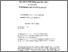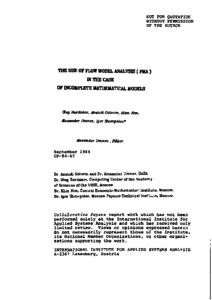# The Use of Flow Model Analysis (FMA) in the Case of Incomplete Mathematical Models

Burdakov, O., Golovin, A., Kim, K., Umnov, A., & Shompolov, I. (1984). The Use of Flow Model Analysis (FMA) in the Case of Incomplete Mathematical Models. IIASA Collaborative Paper. IIASA, Laxenburg, Austria: CP-84-040Preview Text CP-84-040.pdf Download (428kB) | Preview

## Abstract

The main aim of the "System for Analyzing Mathematical Flow Models" (FMA system) described in this paper is to supply the decision-maker with a computerized tool for quantitative investigation of problem that can be described, at least partially, in terms of standard mathematical models of the Bow type.

The FMA system permits the decision-maker to find states of the considered model that satisfy all introduced constraints, are close to the desirable structure, and are optimal with respect to single- or multiobjective evaluation.

Item Type: Monograph (IIASA Collaborative Paper) Regional Issues Program (RUD) IIASA Import 15 Jan 2016 01:55 27 Aug 2021 17:12 https://pure.iiasa.ac.at/2537View Item